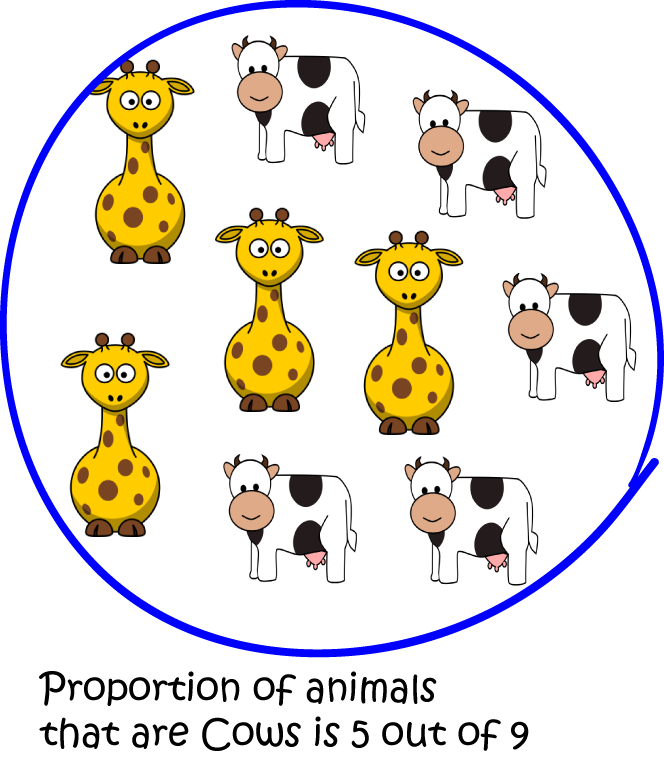# Definition of ProportionA proportion is the ratio of the number of objects in a set having some property to the total number of objects in the set.

For example, $5$ of the $9$ animals in the pictured set are cows. So, the proportion of cows is $5$ out of $9$, which can be written as the ratio $5:9$.

Proportion is also used as another term for ratio.

In the US, the term proportion is used to mean an equation which says that two fractions or ratios are equal. An example is $\dfrac{x}{5} = \dfrac{8}{20}$.

### Description

The aim of this dictionary is to provide definitions to common mathematical terms. Students learn a new math skill every week at school, sometimes just before they start a new skill, if they want to look at what a specific term means, this is where this dictionary will become handy and a go-to guide for a student.

### Audience

Year 1 to Year 12 students

### Learning Objectives

Learn common math terms starting with letter P

Author: Subject Coach
You must be logged in as Student to ask a Question.